Register for Maths, Science, English, Reasoning Olympiad Exams Register here | Book Free Demo for Live Olympiad Classes here | Check Olympiad Exam Dates here | Buy Practice Papers for IMO, IOM, HEO, IOEL etc here | Login here to participate in all India free mock test on every Saturday

# Measurements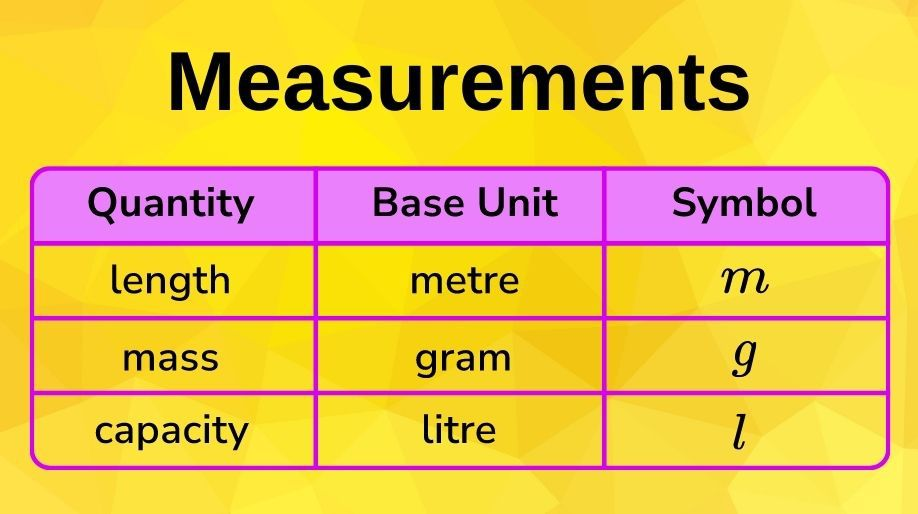• If we consider metre, litre, and gram as units of measurement, the higher units are obtained by adding the prefixes deca (meaning ten), hecto (meaning hundred) and kilo (meaning thousand).
• The lower units are obtained by adding the prefixes deci (meaning tenth), centi (meaning hundredth), milli (meaning thousandth)

 Thousands 1000 Hundreds 100 Tens 10 Ones Tenths 1/10 Hundredths 1/100 Thousandth 1/1000 Kilo Hecto Deca Metre Litre Gram Deci Centi Milli

# Measures of length

Length is the longest extent of anything as measured from end to end.

Length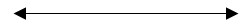In the above example length is
used to measure how long the line is.

• 10 millimetres (mm) = 1 centimetre
• 10 centimetres (cm) = 1 decimetre
• 10 decimetres (dm) = 1 metre
• 10 metres (m) = 1 decametre
• 10 decametres (dam) = 1 hectometre
• 10 hectometres (hm) = 1 kilometre (km)

# Measures of mass

Mass is the measure of amount of matter in an object.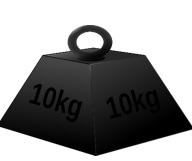• 10 milligrams (mg) = 1 centigram
• 10 centigrams (cg) = 1 decigram
• 10 decigrams (dg) = 1 gram
• 10 grams (g) = 1 decagram
• 10 decagrams (dag) = 1 hectogram
• 10 hectograms (hg) = 1 kilogram (kg)

Trivia Question:

How many centimetres in a mile?

# Measures of capacity

Capacity is the amount a container can hold

• 10 millilitres (ml) = 1 centilitre
• 10 centilitres (cl) = 1 decilitre
• 10 decilitres (dl) = 1 litre
• 10 litres (l) = 1 decalitre
• 10 decalitres (dal) = 1 hectolitre
• 10 hectolitres (hl) = 1 kilolitre (kl)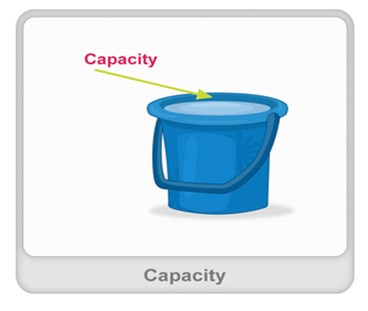# Measures of volume

Volume is the measure of the space taken up by something.

• 1000 cubic millimetres (mm3) = 1 cubic centimetre
• 1000 cubic centimetres (cm3) = 1 cubic decimetre
• 1000 cubic decimetres (dm3) = 1 cubic metre
• 1000 cubic metres (m3) = 1 cubic decametre
• 1000 cubic decametres (dam3) = 1 cubic hectometre
• 1000 cubic hectometres (hm3) = 1 cubic kilometre (km3)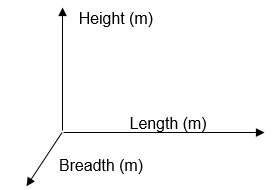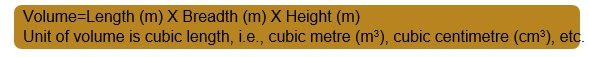EXAMPLE 1: Express 1357.912 metres in higher units and lower units.

SOLUTION:

 Kilometre Hectometre Decametre Metre Decimetre Centimetre Millimetre 1 3 5 7 9 1 2

1357.912 metre

=135.7912 decametres

=13.57912 hectometres

=1.357912 kilometres

=13579.12 decimetres

=135791.2 centimetres

=1357912 millimetres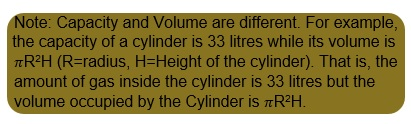EXAMPLE 2: Express 2 g 3 dg 4cg 5mg in terms of milligrams.

SOLUTION:

2g = 2000mg

3dg= 300mg

4cg= 40mg

2g 3dg 4cd 5mg = (2000 + 300 + 40 + 5) mg =2345mg [Ans]

EXAMPLE 3: Express 1m3 6dm3 7cm3 8mm3 in terms of mm3.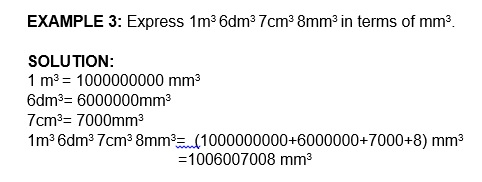• Add the numbers in each position, if a carry is generated then add the carry with the digits in the next position.

EXAMPLE 1: Add 2l 3dl 4cl 5ml & 6l 6dl 7cl 8ml

SOLUTION:

2l 3dl 4cl 5ml + 6l 6dl 7cl 8ml 9l 0dl 2cl 3ml [Ans]

EXAMPLE 2: Express the above result in terms of ml.

SOLUTION:

2345ml + 6678ml 9023ml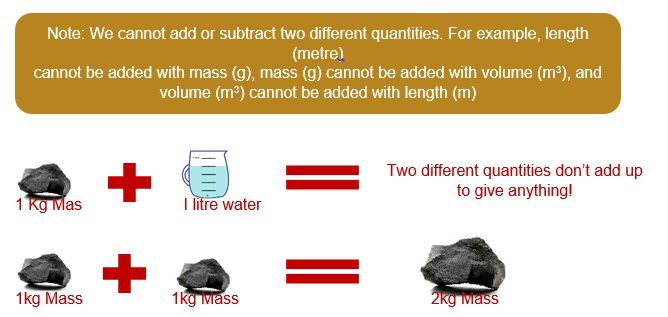• Subtract the number in each position, if a borrow is taken then add it to the next position where subtraction will take place.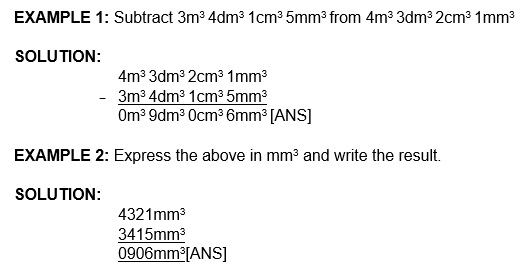# Measurements: Multiplication and Division

• When a quantity is multiplied by a number, we are actually adding the same quantity a repeated number of times.
For example,
When 15kg is multiplied
by 3, we are actually adding
the amount 15kg three times.
• When a quantity is divided by a number, we are actually splitting the quantity.
For example,
6 litres of oil is
to be divided into
3 separate containers.
Here, we are dividing 6 by 3.
which is equal to 2. Thus, each
container would contain 2 litres of oil.
• Two different quantities can be multiplied or divided. When we do so, we get a new quantity.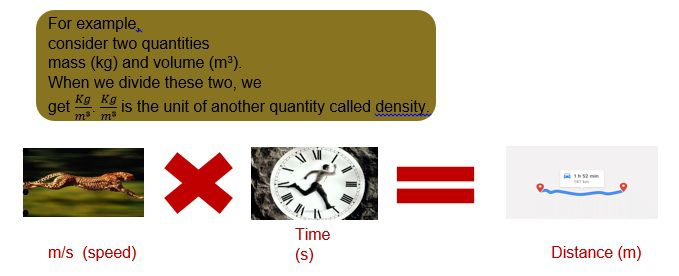EXAMPLE 1: Multiply 123g by 23.

SOLUTION:

1 2 3 g
X2 3_
369
+2460
2829 g [ANS]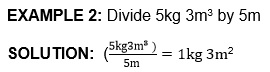# Temperature

• Temperatureis the measure of the hotness or coldness of a substance. A thermometer is an instrument which is used to measure temperature.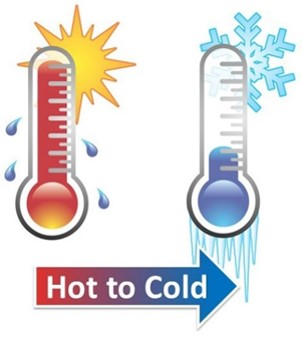• Temperature is measured in three scales: Celsius, Fahrenheit, and Kelvin.
 From To Fahrenheit To Celsius Fahrenheit (F) F (F - 32) X 5/9 Celsius (C) (CX9/5) + 32 C Kelvin (K) (K-273.15) X 9/5 +32 K-273.15

Fahrenheit Scale- A temperature scale on which water freezes at 32 degrees Fahrenheit and boils at 212 degrees Fahrenheit.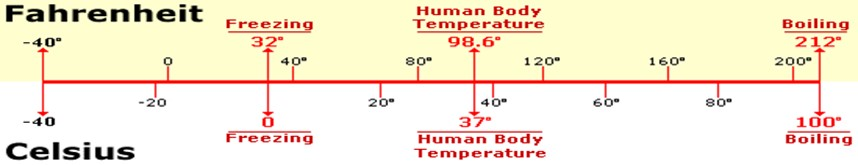Kelvin Scale: A temperature scale that defines absolute zero as 0 degrees. Water freezes at 273 degrees and boils at 373 degrees at this temperature.
All objects emit thermal energy or heat unless they have a temperature of absolute zero.

EXAMPLE 1: Convert 123 degrees Fahrenheit into Celsius and Kelvin.

SOLUTION:

Celsius= (F-32) X 5/9 = (123-32) X 5/9 =50.55 degree Celsius Kelvin= Celsius + 273 = 50.55 + 273 = 323.55 K

EXAMPLE 2: Convert 45 Celsius into Fahrenheit and kelvin.

SOLUTION:

Fahrenheit= (CelsiusX9/5) + 32 = (45X9/5) + 32 =113 degrees Fahrenheit Kelvin=Celsius + Fahrenheit = 45+273 = 318 K

# Practice these questions

Q1) A bus travels 123km 45m in 6 hours. Give in km the distance travelled by the bus in 1 hour.

Q2) 7m 89cm of cloth is required to stitch 1 dress. Find the length of cloth needed in metres to stitch 23 dresses.

Q3) There was 4L 56mL of coffee in a pot. It was distributed among 7 people, each getting 89ml of coffee. While distributing, 1201ml of coffee fell on the floor. How much coffee is left in the pot?

Q4) 123kg=_____hg=_____ dag =_____g

Q5) Find the total boundary of the box given below: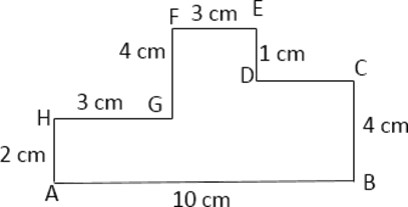Q6) Convert 67 K into Fahrenheit and Celsius.

# Recap

• Two quantities can be multiplied or divided but cannot be added or subtracted.
• Length is the longest extent of anything.
• Mass is the measure of amount of matter.
• Capacity & Volume are different.
• Temperature measures how hot or cold a substance is.
• A thermometer is used to measure temperature.
• Temperature can be measured in three scales: Fahrenheit, Celsius, and Kelvin.

## Quiz for Measurements

 Q.1 Fill in the blank. 3 hm 9 dam = _____ a) 310 m b) 390 m c) 410 m d) 385 m
 Q.2 Fill in the blank. 8 dam = _____ a) 80,000 cm b) 80 cm c) 8000 cm d) 800 cm
 Q.3 Fill in the blank. 4 km 3 hm 5 dam 4 m = _____ a) 40354 m b) 3454 m c) 484 m d) 4354 m
 Q.4 Fill in the blank. 3.6 km = _____ a) 360 dam b) 3600 dam c) 36 dam d) 36000 dam
 Q.5 Rajesh ran in a park in 3 slots. In the first slot, he ran 20 m 30 cm. In the second slot he ran 63 m and in the third slot, he ran 5000 cm. Total how long did he run? a) 13330 cm b) 83330 cm c) 9430 cm d) 12230 cm
 Q.6 Steven runs half a kilometre per day. How many centimetres does he run in 3 days? a) 350000 b) 15000 c) 150000 d) 35000
 Q.7 Rohan travelled 200 km in 4 days. How many metres did he travel in a day? a) 500000 m b) 5000 m c) 25000 m d) 50000 m
 Q.8 I have 3 pencils. Each pencil is 120 millimetres long. What is the length of pencil in metre? a) 0.36 m b) 0.12 m c) 3.6 m d) 1.2 m
 Q.9 Radha bought 20 m long velvet but she used only 11 m 75 cm out of it. How much remains unused? a) 975 cm b) 825 cm c) 750 cm d) 925 cm
 Q.10 Fill in the blank. 7 km 5 hm 6 m = _____ a) 75600 cm b) 7056 m c) 7560 m d) 750600 cm